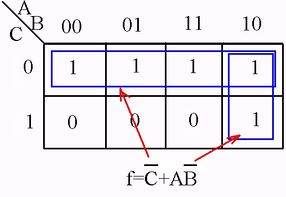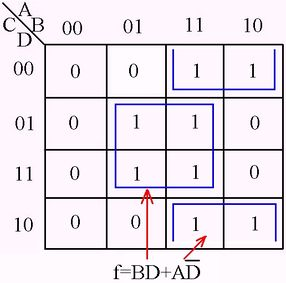# Introduction

Logic design implies the analysis, synthesis, minimization, and implementation of binary functions. Combinational logic refers to networks whose output is strictly dependent on the inputs. The analysis of such networks requires first the writing of the Boolean algebraic equation representative of the network, and then the complete characterization of the output as a result of all the possible combinations of the inputs. Minimization involves reducing the Boolean algebraic expression to some minimal form.
Any minimization tool in Boolean is based on the algebraic theorems. Algebraic reduction of Boolean functions is not easy and requires considerable experience, judgement and luck. It becomes more apparent as the complexity of the function increases.

For example the two input variable expression as $A \overline{B} + \overline{A} \overline{B}$ is obviously dependent only on $\overline{B}$. But it is not so obvious that the following three input expression $A \overline{B} + \overline{B} C + \overline{A} C$ is represented by $A \overline{B} + \overline{A} C$ .

As a result extensive effort has been devoted toward developing techniques, aids or tools, that will allow the logic designer to minimize a function. The Venn diagram, Veitch diagram, Karnaugh map, Quine-McCluskey method, and other techniques have all been developed with but one objective-to allow the designed to arrive at a minimal expression as rapidly as possible with the least amount of effort.

Among these methods the Karnaugh Maps (made by G. Karnaugh in 1953) will be presented bellow.

The Karnaugh map is an orderly arrangement of squares with assignments such that the difference between any two adjacent squares is a one-variable change. The map must contain a square or cell for each unique combination of input variables. A map for two variables A and B must have four cells, because there are 22 different combinations of two input variables. A map for three variables A, B and C must contain 23 or eight cells, and a map on n variables must contain 2n cells. An assignment of 1 for uncomplemented variable and 0 for a complemented variable is made. For instance, a term $A \overline{B} C$ is equivalent to the binary notations 101. The table bellow shows the ways to define each cell of two, three and four variables functions.
The alphabetical input variables are identified at the upper left-hand corner of the box. For example, for three input variable function, the diagonal lines indicates that the variables A and B are represented by the binary notations across the top of the matrix (which is a Gray Code with the unique property of only a single bit change when going from one state to the next) and are contained in the vertical column below each assignment. The variable C is designed down the side of the matrix and is represented by horizontal rows within the matrix. Each cell represents a unique combination of the variables.

The Karnaugh map technique is thought to be the most valuable tool available for dealing with Boolean functions. It provides instant recognition of the basic patterns, can be used to obtain all possible combinations and minimal terms, and is easily applied to all varieties of complex problems. Minimization with the map is accomplished through recognition of basic patterns.
The appearance of 1’s in adjacent cells immediately identifies the presence of a redundant variable.
The following table illustrates some examples of minimizing with two, three, four and five-variable map.

This table illustrates the grouping of one, two, four, eight and sixteen cells, depending on the number of inputs. For example, in a four-variable map, the grouping of two cells eliminates one variable, the grouping of four cells eliminates two variables, and the grouping of eight cells eliminates three variables. The rule is the same for different number of input variables.
Any given grouping of 1’s in the Karnaugh map is identified by a product form.
Minimization involves the gathering of the various groups in the most efficient manner. The mapping of a sum of product terms is accomplished by inserting a 1 in each cell of a map containing a particular product term.
The advantage of the map is that it gives a clear and complete picture of the function. It can immediately be determined if the reduction is unique or if there exist other minimal expressions.

# Karnaugh Map examples

RULES SUMMARY

No zeros allowed.

No diagonals.

Only power of 2 number of cells in each group.

Groups should be as large as possible.

Every one must be in at least one group.

Overlapping allowed.

Wrap around allowed.

Fewest number of groups possible.

# Sample problems

## Sample problem 1

Karnaugh Map to minimize a digital circuit

## Sample problem 2

Karnaugh Map to minimize a digital circuit

## Sample problem 3

Karnaugh Map to minimize a digital circuit

## Sample problem 4

Simplify the following Boolean three variable equation using Karnaugh map.

Initial function Karnaugh Map Minimal function
$f = \overline{A} \overline{B} \overline{C} + A B \overline{C} +A \overline{B} + \overline{A} \overline{C}$$f = \overline{C} + A \overline{B}$

## Sample problem 5

Simplify the following Boolean four variable equation using Karnaugh map.

Initial function Karnaugh Map Minimal function
$f = A \overline{B} \overline{D} + A B \overline{C} \overline{D} + A B C + B \overline{C} D + \overline{A} B C D$$f = B D + A \overline{D}$

1. William E. Wickes, Logic Design with Integrated Circuits, John Willey & Sons, Inc, New York-London-Sydney